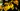# 你知道 +0 与 -0 的区别吗？

JavaScript 的数值 Number 用 64 位的浮点数表示，首位是符号位，然后是 52 位的整数位和 11 位的小数位。如果符号位为 1，其他各位均为 0，那么这个数值会被表示成 `-0`

``````const num = -0;
console.log(num); // -0``````

``````// 首先创建一个8位的ArrayBuffer
const buffer = new ArrayBuffer(8);
// 创建DataView对象操作buffer
const dataView = new DataView(buffer);

// 将第1个字节设置为0x80，即最高位为1
dataView.setUint8(0, 0x80);

// 将buffer内容当做Float64类型返回
console.log(dataView.getFloat64(0)); // -0``````

``````console.log(1 / -Infinity); // -0
console.log(-1 / Infinity); // -0
console.log(-Number.MIN_VALUE / 2); // -0
console.log(-1e-1000); // -0``````

``````console.log(-0 === 0); // true

const num = -0;
if (!num) {
// !num 为 true
// do sth.
}``````

``````const num = -0;

console.log(1 / num > 0); // false
console.log(Math.sign(1 / num)); // -1``````

`-0` 也有好处，我们可以利用 `-0` 来表示状态，比如我们在寻路的时候，把地图的障碍物用 1 表示，空地用 0 表示，那么我们就可以把未搜索过的用 -1 和 `-0` 表示，这样也挺方便的。

`num === -0` 是不行的，因为 `0 === -0` 也会返回 `true`

`Math.sign` 也是不行的，`Math.sign` 一共会返回 4 种值：

``````console.log(Math.sign(0)); // 0
console.log(Math.sign(-0)); // -0
console.log(Math.sign(0.01)); // 1
console.log(Math.sign(-0.01)); // -1``````

👉🏻 【冷知识】`Object.is(value1, value2)` 比较两个值是否相同。它与 `===` 的区别是，`Object.is(-0, 0)` 返回 `false``Object.is(NaN, NaN)` 返回 `true`

``````function isNegativeZero(num) {
return num === 0 && 1 / num < 0;
}``````

``````if (!Object.is) {
Object.is = function (x, y) {
// SameValue algorithm
if (x === y) {
// Steps 1-5, 7-10
// Steps 6.b-6.e: +0 != -0
return x !== 0 || 1 / x === 1 / y;
} else {
// Step 6.a: NaN == NaN
return x !== x && y !== y;
}
};
}``````